Home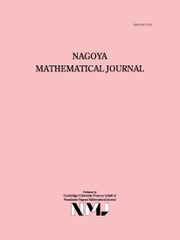Nagoya Mathematical Journal

# TORSION OF ABELIAN VARIETIES OVER LARGE ALGEBRAIC EXTENSIONS OF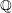$\mathbb{Q}$

Published online by Cambridge University Press:  10 November 2017

Corresponding

## Abstract

Let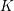$K$ be a finitely generated extension of$\mathbb{Q}$ , and let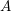$A$ be a nonzero abelian variety over$K$ . Let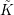$\tilde{K}$ be the algebraic closure of$K$ , and let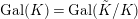$\text{Gal}(K)=\text{Gal}(\tilde{K}/K)$ be the absolute Galois group of$K$ equipped with its Haar measure. For each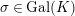$\unicode[STIX]{x1D70E}\in \text{Gal}(K)$ , let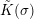$\tilde{K}(\unicode[STIX]{x1D70E})$ be the fixed field of$\unicode[STIX]{x1D70E}$ in$\tilde{K}$ . We prove that for almost all$\unicode[STIX]{x1D70E}\in \text{Gal}(K)$ , there exist infinitely many prime numbers$l$ such that$A$ has a nonzero$\tilde{K}(\unicode[STIX]{x1D70E})$ -rational point of order$l$ . This completes the proof of a conjecture of Geyer–Jarden from 1978 in characteristic 0.

## MSC classification

Type
Article
Information
Nagoya Mathematical Journal , June 2019 , pp. 46 - 86
© 2017 Foundation Nagoya Mathematical Journal

## Access options

Get access to the full version of this content by using one of the access options below.

## References

Borel, A., Linear Algebraic Groups, 2nd enlarged edn., Graduate Texts in Mathematics 126 , Springer, New York, 1991.10.1007/978-1-4612-0941-6CrossRefGoogle Scholar
Cadoret, A., An open adelic image theorem for abelian schemes , Int. Math. Res. Not. IMRN 20 (2015), 1020810242.10.1093/imrn/rnu259CrossRefGoogle Scholar
Cadoret, A. and Tamagawa, A., A uniform open image theorem for l-adic representations , Duke Math. J. 161 (2012), 26052634.10.1215/00127094-1812954CrossRefGoogle Scholar
Cadoret, A. and Tamagawa, A., A uniform open image theorem for l-adic representations II , Duke Math. J. 162 (2013), 23012344.10.1215/00127094-2323013CrossRefGoogle Scholar
Eisenbud, D., Commutative Algebra with a View Toward Algebraic Geometry, Graduate Texts in Mathematics 150 , Springer, New York, 1995.Google Scholar
Engler, A. J. and Prestel, A., Valued Fields, Springer, Berlin, Heidelberg, 2010.Google Scholar
Fried, M. D. and Jarden, M., Field Arithmetic, 3rd edn., Ergebnisse der Mathematik (3) 11 , Springer, Heidelberg, 2008, Revised by Moshe Jarden.Google Scholar
Gajda, W. and Petersen, S., Independence of l-adic Galois representations over function fields , Compositio Math. 149 (2013), 10911107.CrossRefGoogle Scholar
Geyer, W.-D. and Jarden, M., Torsion points of elliptic curves over large algebraic extensions of finitely generated fields , Israel J. Math. 31 (1978), 157197.Google Scholar
Geyer, W.-D. and Jarden, M., Torsion of Abelian varieties over large algebraic fields , Finite Field Theory Appl. 11 (2005), 123150.10.1016/j.ffa.2004.02.004CrossRefGoogle Scholar
Görtz, U. and Wedhorn, T., Algebraic Geometry I, Vieweg + Teubner, Wiesbaden, 2010.10.1007/978-3-8348-9722-0CrossRefGoogle Scholar
Grothendieck, A., Éléments de Géométrie Algébrique IV, seconde partie , Publ. Math. Inst. Hautes Études Sci. 24 (1965), 5231.Google Scholar
Grothendieck, A., Éléments de Géométrie Algébrique IV, troisième partie , Publ. Math. Inst. Hautes Études Sci. 28 (1966), 5255.CrossRefGoogle Scholar
Grothendieck, A., Revêtement étales et groupe fondamental (SGA 1), Lecture Notes in Mathematics 224 , Springer, Berlin, 1971.Google Scholar
Hartshorne, R., Algebraic Geometry, Graduate Texts in Mathematics 52 , Springer, New York, 1977.10.1007/978-1-4757-3849-0CrossRefGoogle Scholar
Jacobson, M. and Jarden, M., On torsion of abelian varieties over large algebraic extensions of finitely generated fields , Mathematika 31 (1984), 110116.10.1112/S0025579300010718CrossRefGoogle Scholar
Jacobson, M. and Jarden, M., On torsion of abelian varieties over large algebraic extensions of finitely generated fields: erratum , Mathematika 32 (1985), 316.10.1112/S0025579300011098CrossRefGoogle Scholar
Jacobson, M. and Jarden, M., Finiteness theorems for torsion of abelian varieties over large algebraic fields , Acta Arith. 98 (2001), 1531.10.4064/aa98-1-2CrossRefGoogle Scholar
Lang, S., Introduction to Algebraic Geometry, Interscience Publishers, New York, 1958.Google Scholar
Liu, Q., Algebraic Geometry and Arithmetic Curves, Oxford Graduate Texts in Mathematics 6 , Oxford University Press, Oxford, 2006.Google Scholar
Milne, J. S., Étale Cohomology, Princeton University Press, Princeton, 1980.Google Scholar
Milne, J. S., “ Abelian varieties ”, in Arithmetic Geometry (eds. Cornell, G. and Silverman, J. H.) Springer, New York, 1985.Google Scholar
Mumford, D., Abelian Varieties, Oxford University Press, London, 1974.Google Scholar
Mumford, D., The Red Book of Varieties and Schemes, Lecture Notes in Mathematics 1358 , Springer, Berlin, 1988.CrossRefGoogle Scholar
Raynaud, M., Anneaux Locaux Henséliens, Lecture Notes in Mathematics 169 , Springer, Berlin, 1970.CrossRefGoogle Scholar
Serre, J.-P., “ Zeta and L-functions ”, in Arithmetical Algebraic Geometry (ed. Schilling) Harper and Row, New York, 1965, 8292.Google Scholar
Serre, J.-P., Groupes linéaires modulo$p$ et points d’ordere fini des varietés abéliennes, Note of a cours at Collège de France given in 1986, taken by Eva Bayer-Flukiger.Google Scholar
Serre, J.-P., Œuvres. Collected Papers, IV, 1985–1998, Springer, Berlin, 2000.Google Scholar
Serre, J.-P., Un critère d’indépendance pour une famille de représentation l-adiques , Comment. Math. Helv. 88 (2013), 451554.10.4171/CMH/295CrossRefGoogle Scholar
Serre, J.-P. and Tate, J., Good reduction of abelian varieties , Ann. of Math. (2) 88 (1968), 492571.10.2307/1970722CrossRefGoogle Scholar
Shimura, G., Abelian Varieties with Complex Multiplication and Modular Functions, Princeton University Press, Princeton, 1998.10.1515/9781400883943CrossRefGoogle Scholar
Springer, T. A., Linear Algebraic Groups, 2nd edn. Birkhäuser, Boston, 1998.10.1007/978-0-8176-4840-4CrossRefGoogle Scholar
Tate, J., Endomorphisms of Abelian varieties over finite fields , Invent. Math. 2 (1966), 134144.10.1007/BF01404549CrossRefGoogle Scholar
Zywina, D., Abelian varieties over large algebraic fields with infinite torsion , Israel J. Math. 211 (2016), 493508.10.1007/s11856-015-1268-3CrossRefGoogle Scholar

### Full text views

Full text views reflects PDF downloads, PDFs sent to Google Drive, Dropbox and Kindle and HTML full text views.

Total number of HTML views: 12
Total number of PDF views: 82 *
View data table for this chart

* Views captured on Cambridge Core between 10th November 2017 - 18th January 2021. This data will be updated every 24 hours.

Hostname: page-component-77fc7d77f9-qmqs2 Total loading time: 0.552 Render date: 2021-01-18T05:11:22.629Z Query parameters: { "hasAccess": "0", "openAccess": "0", "isLogged": "0", "lang": "en" } Feature Flags last update: Mon Jan 18 2021 05:03:48 GMT+0000 (Coordinated Universal Time) Feature Flags: { "metrics": true, "metricsAbstractViews": false, "peerReview": true, "crossMark": true, "comments": true, "relatedCommentaries": true, "subject": true, "clr": true, "languageSwitch": true, "figures": false, "newCiteModal": false, "shouldUseShareProductTool": true, "shouldUseHypothesis": true, "isUnsiloEnabled": true }

# Send article to Kindle

Note you can select to send to either the @free.kindle.com or @kindle.com variations. ‘@free.kindle.com’ emails are free but can only be sent to your device when it is connected to wi-fi. ‘@kindle.com’ emails can be delivered even when you are not connected to wi-fi, but note that service fees apply.

Find out more about the Kindle Personal Document Service.

TORSION OF ABELIAN VARIETIES OVER LARGE ALGEBRAIC EXTENSIONS OF$\mathbb{Q}$Available formats × # Send article to Dropbox To send this article to your Dropbox account, please select one or more formats and confirm that you agree to abide by our usage policies. If this is the first time you use this feature, you will be asked to authorise Cambridge Core to connect with your <service> account. Find out more about sending content to Dropbox. TORSION OF ABELIAN VARIETIES OVER LARGE ALGEBRAIC EXTENSIONS OF$\mathbb{Q}$
Available formats
×

# Send article to Google Drive

To send this article to your Google Drive account, please select one or more formats and confirm that you agree to abide by our usage policies. If this is the first time you use this feature, you will be asked to authorise Cambridge Core to connect with your <service> account. Find out more about sending content to Google Drive.

TORSION OF ABELIAN VARIETIES OVER LARGE ALGEBRAIC EXTENSIONS OF$\mathbb{Q}\$
Available formats
×
×